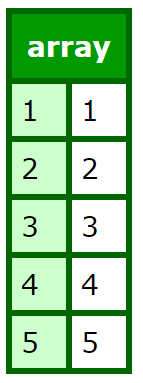Array elements can store any values, including queries, structures, and other arrays. You can use assignment statements to populate an array. You can also use several of functions to populate an array with data, including ArraySetArrayAppendArrayInsertAt, and ArrayPrepend. These functions are useful for adding data to an existing array.

In particular, consider using the following techniques:

• Populating an array with the ArraySet function
• Populating an array with the cfloop tag
• Populating an array from a query

### Populating an array with the ArraySet function

You can use the ArraySet function to populate a 1D array, or one dimension of a multidimensional array, with some initial value, such as an empty string or zero. This can be useful to create an array of a certain size, without adding data to it right away. One reason to do this is so that you can reference all the array indexes. If you reference an array index that does not contain some value, such as an empty string, you get an error.
The ArraySet function has the following form:

`ArraySet (arrayname, startrow, endrow, value)`

The following example initializes the array myarray, indexes 1 - 100, with an empty string:

`ArraySet (myarray, 1, 100, "")`

### Populating an array with the cfloop tag

The cfloop tag provides a common and efficient method for populating an array. The following example uses a cfloop tag and the MonthAsString function to populate a simple 1D array with the names of the months. A second cfloop outputs data in the array to the browser.

```<cfloop index="loopcount" from=1 to=12>
<cfset months[loopcount]=MonthAsString(loopcount)>
</cfloop>

<cfloop index="loopcount" from=1 to=12>
<cfoutput>
#months[loopcount]#<br>
</cfoutput>
</cfloop>```

### Using nested loops for 2D and 3D arrays

To output values from 2D and 3D arrays, employ nested loops to return array data. With a one-dimensional (1D) array, a single cfloop is sufficient to output data, as in the previous example. With arrays of dimension greater than one, you maintain separate loop counters for each array level.

### Nesting cfloop tags for a 2D array

The following example shows how to handle nested cfloop tags to output data from a 2D array. It also uses nested cfloop tags to populate the array:

```<cfloop index="loopcount" from=1 to=12>
<cfloop index="loopcount2" from=1 to=2>
<cfset my2darray[loopcount][loopcount2]=(loopcount * loopcount2)>
</cfloop>
</cfloop>

<p>The values in my2darray are currently:</p>

<cfloop index="OuterCounter" from="1" to="#ArrayLen(my2darray)#">
<cfloop index="InnerCounter" from="1"to="#ArrayLen(my2darray[OuterCounter])#">
<cfoutput>
<b>[#OuterCounter#][#InnerCounter#]</b>:
#my2darray[OuterCounter][InnerCounter]#<br>
</cfoutput>
</cfloop>
</cfloop>```

### Nesting cfloop tags for a 3D array

For 3D arrays, you simply nest an additional cfloop tag. (This example does not set the array values first to keep the code short.)

```<cfloop index="Dim2" from="1" to="#ArrayLen(my3darray[Dim1])#">
<cfloop index="Dim3" from="1"to="#ArrayLen(my3darray[Dim1][Dim2])#">
<cfoutput>
<b>[#Dim1#][#Dim2#][#Dim3#]</b>:
#my3darray[Dim1][Dim2][Dim3]#<br>
</cfoutput>
</cfloop>
</cfloop>
</cfloop>```

### Populating an array from a query

When populating an array from a query, remember the following:

• You cannot add query data to an array all at once. A looping structure is often required to populate an array from a query.
• You can reference query column data using array-like syntax. For example, myquery.col_name1 references data in the first row in the col_name column of the myquery query.
• Inside a cfloop query= loop, you do not have to specify the query name to reference the query variables.
You can use a cfset tag with the following syntax to define values for array indexes:
```<cfset arrayName[index]=queryColumn[row]>

In the following example, a cfloop tag places four columns of data from a sample data source into an array, myarray.

<cfquery name="test" datasource="cfdocexamples">
SELECT Emp_ID, LastName, FirstName, Email
FROM Employees
</cfquery>

<!--- Declare the array --->
<cfset myarray=arraynew(2)>

<!--- Populate the array row by row --->
<cfloop query="test">
<cfset myarray[CurrentRow]=Emp_ID>
<cfset myarray[CurrentRow]=LastName>
<cfset myarray[CurrentRow]=FirstName>
<cfset myarray[CurrentRow]=Email>
</cfloop>

<!--- Now, create a loop to output the array contents --->
<cfset total_records=test.recordcount>
<cfloop index="Counter" from=1 to="#Total_Records#">
<cfoutput>
ID: #MyArray[Counter]#,
LASTNAME: #MyArray[Counter]#,
FIRSTNAME: #MyArray[Counter]#,
EMAIL: #MyArray[Counter]# <br>
</cfoutput>
</cfloop>```

This example uses the query object built-in variable CurrentRow to index the first dimension of the array.

### Typed arrays

The 2018 release of ColdFusion  supports declaring arrays and structs with a set of related types.

In previous versions of ColdFusion, you could create an array with the following code:

```<cfscript>
arr=arrayNew() // or arr=[];
arr=["hello","world"];
writeDump(arr);
</cfscript>
```

Using type, you can now rewrite the above as:

```<cfscript>
arr=arrayNew["String"](1);
arr.append("hello");
arr.append("world");
writeDump(arr);
</cfscript>
```

The output is an array of strings.

The supported types are:

• String
• Numeric
• Boolean
• Date / Datetime
• Array
• Struct
• Query
• Component
• CFC (By Name / Subtype)
• Binary
• Function

Note:

The type declaration allows you to insert data of only the declared type. For more information on data types, see Data types developing guide.

In addition, there is support for:

• Inheritance while inserting CFCs
• Function getType to fetch the type contained in the typed array
• Typed arrays in method argument (for example, numeric function getMax(numeric[] numbers))
• Typed arrays in method return type (for example, Student[] function getStudents(numeric[] studentIds))

### Slicing in arrays

In ColdFusion, to slice an array means extracting elements from an array depending on a start and stop.

For example, in the following script,

```<cfscript>
a=[1,2,3,4,5,6,7,8]
writedump(a[1:6])
</cfscript>
```

OutputSimilarly,

```<cfscript>
a=[1,2,3,4,5,6,7,8]
writedump(a[1:6:2]) // In steps of 2
</cfscript>
```

The usage for step in the slicing of an array is Array[start : end : step]

```<cfscript>
a=[1,2,3,4,5,6,7,8]
writedump(a[5:]) // from index 5 till the end of the array
</cfscript>
```
```<cfscript>
a=[1,2,3,4,5,6,7,8]
writedump(a[:3]) // All elements till index 3, exclusive of index 3
</cfscript>
```
```<cfscript>
a=[1,2,3,4,5,6,7,8]
writedump(a[:]) // returns the entire array
</cfscript>
```

Using negative indices,

```<cfscript>
a=[1,2,3,4,5,6,7,8]
writedump(a[:-5]) // Returns all elements from the array, except the last 5 elements
</cfscript>
```
```<cfscript>
a=[1,2,3,4,5,6,7,8]
writedump(a[:-2:2])
</cfscript>
```
```<cfscript>
values = ["Aa","Bb","Cc","Dd","Ee"]
odds = values[::2]
writedump(odds)
</cfscript>
```Twitter™ and Facebook posts are not covered under the terms of Creative Commons.